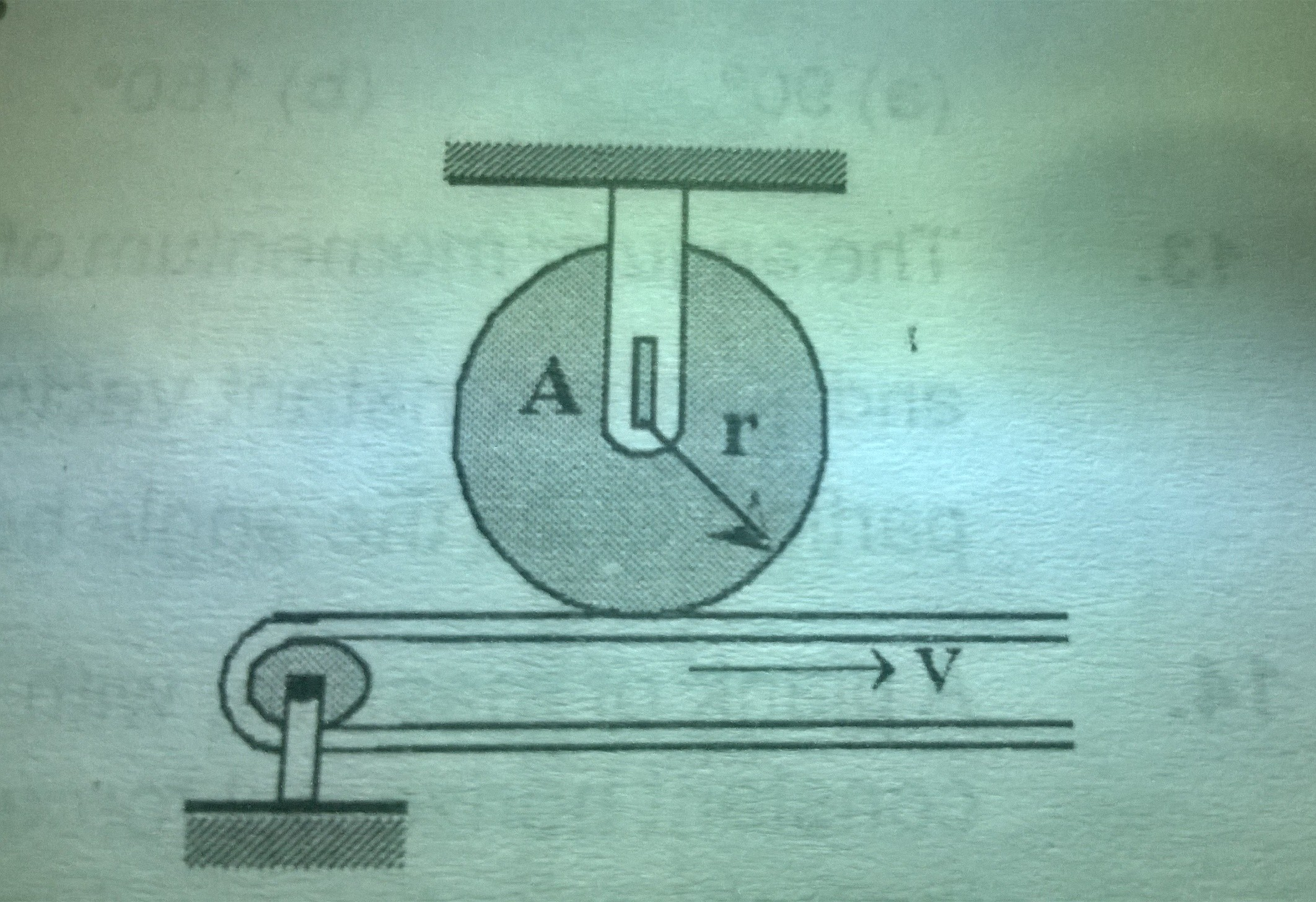# A good rotational motion question!Disc A has a mass of 4 kg and a radius $r=75$ mm ,it is at rest when it is placed in contact with the belt, which moves at a constant speed $v=18$ ms$^{-1}$ . Knowing that coefficient of kinetic friction is $u_{k}=$0.25 between the disc and the belt , determine the number of revolutions executed by the disc before it reaches a constant angular velocity Assume that the normal reaction by the belt on the disc is equal to weight of the disc.

×

Problem Loading...

Note Loading...

Set Loading...